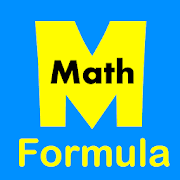# All Math Formula - Lumos Educational App Store4.31
Price -fREE
\$0

#### DESCRIPTION:

Apps containing most of the essentials math formula and equations under the following categories- * Algebra * Geometry * Analytical Geometry * Trigonometry * Derivatives * Integrals * Limits * Vector Calculus * Series * Probability * Laplace Transform * Fourier Series * Basic Math Symbols * Greek Alphabet Details: * Algebra - Basic Properties - Absolute Value Inequalities - Arithmetic Operations - Complex Numbers - Distance Formula - Exponent Properties - Inequality Properties - Logarithm Properties - Radical Properties - Zero Value Properties - Factoring and

#### OVERVIEW:

All Math Formula is a free educational mobile app By .It helps students in grades HS practice the following standards HSF.TF.C.9.

This page not only allows students and teachers download All Math Formula but also find engaging Sample Questions, Videos, Pins, Worksheets, Books related to the following topics.

1. HSF.TF.C.9 : Prove the addition and subtraction formulas for sine, cosine, and tangent and use them to solve problems..

HS

HSF.TF.C.9

#### ADDITIONAL INFORMATION:

Developer:

Software Version: 1.1

Category:

### RELATED APPSEdSearch WebSearch